cancel
Showing results for
Did you mean: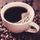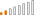Continued Contributor

## Trying to create measure with this logic

I am trying to create a measure based on this logic, and need your advice.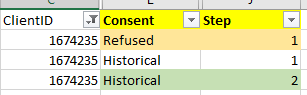Basically, I need to specify four criteria:

I am trying to ask whether there is case that meet  these four conditions:

( Step=1 && Consent = "Refused" )  with

( Step=2 && Consent = "Historical")

Depending on YES or NO, it iwll go to other measure to calculate:

I have a screenshot of the measure which I tried to create those four criteria.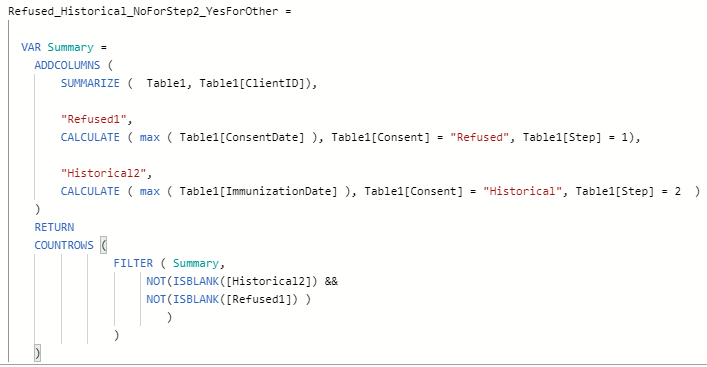Refused_Historical_NoForStep2_YesForOther =
VAR Summary =
SUMMARIZE ( Table1, Table1[ClientID]),
"Refused1",
CALCULATE ( max ( Table1[ConsentDate] ), Table1[Consent] = "Refused", Table1[Step] = 1),
"Historical2",
CALCULATE ( max ( Table1[ImmunizationDate] ), Table1[Consent] = "Historical", Table1[Step] = 2 )
)
RETURN
COUNTROWS (
FILTER ( Summary,
NOT(ISBLANK([Historical2]) &&
NOT(ISBLANK([Refused1]) )
)
)
)

I just used NOT(ISBLANK( ) to count for existence/row.

But, data does not display expected, and I am stuck where to modify.

I am having an issue with creating proper the logic because there are two Steps, instead of one Step.

Should I write like this?

VAR __ClientID = MAX(Table1[ClientID])
VAR __Table1 = FILTER(ALL(Table1),[ClientID]=__ClientID && [Step]=1 && Table1[Consent] = "Refused" )
VAR __Table2 = FILTER(ALL(Table1),[ClientID]=__ClientID && [Step]=2 && Table1[Consent] = "Historical")
VAR __Count1 = COUNTROWS(__Table1)
VAR __Count2 = COUNTROWS(__Table2)
RETURN
IF(__Count1 = 1 && __Count2 = 1 ,1,0)

Thanks for help.

1 ACCEPTED SOLUTIONAnonymous
Not applicable

``````[# Clients with Both Conditions] = // measure, not a calc column
var RefusedCondition =
{("Refused", 1)}
var HistoricalCondition =
{("Historical", 2)}
var Count_ =
SUMX(
DISTINCT( T[ClientID] ),
1 * CALCULATE(
NOT (
ISEMPTY(
FILTER(
T,
( T[Consent], T[Step] )
in RefusedCondition
)
)
||
ISEMPTY(
FILTER(
T,
( T[Consent], T[Step] )
in HistoricalCondition
)
)
)
)
)
return
IF( Count_, Count_ )``````

And here's another formulation of the same measure:

``````[measure_] =
var AttributeSet = {
("Refused", 1),
("Historical", 2)
}
var Count_ =
COUNTROWS(
FILTER(
GROUPBY(
SUMMARIZE(
filter(
T,
( T[Consent], T[Step] )
IN AttributeSet
),
T[ClientID],
T[Consent],
T[Step]
),
T[ClientID],
"@RowCount", SUMX( CURRENTGROUP(), 1 )
),
[@RowCount] = COUNTROWS( AttributeSet )
)
)
return
Count_``````

5 REPLIES 5Anonymous
Not applicable

Because it's always true that:

p and q <=> ~(~p or ~q)Anonymous
Not applicable

``````[# Clients with Both Conditions] = // measure, not a calc column
var RefusedCondition =
{("Refused", 1)}
var HistoricalCondition =
{("Historical", 2)}
var Count_ =
SUMX(
DISTINCT( T[ClientID] ),
1 * CALCULATE(
NOT (
ISEMPTY(
FILTER(
T,
( T[Consent], T[Step] )
in RefusedCondition
)
)
||
ISEMPTY(
FILTER(
T,
( T[Consent], T[Step] )
in HistoricalCondition
)
)
)
)
)
return
IF( Count_, Count_ )``````

And here's another formulation of the same measure:

``````[measure_] =
var AttributeSet = {
("Refused", 1),
("Historical", 2)
}
var Count_ =
COUNTROWS(
FILTER(
GROUPBY(
SUMMARIZE(
filter(
T,
( T[Consent], T[Step] )
IN AttributeSet
),
T[ClientID],
T[Consent],
T[Step]
),
T[ClientID],
"@RowCount", SUMX( CURRENTGROUP(), 1 )
),
[@RowCount] = COUNTROWS( AttributeSet )
)
)
return
Count_``````Continued Contributor

@Anonymous Thank you so much for your help. I have a quick question regards to the 1st query.

On the part where it has ||  (or), would it make difference if I chnage to && for the result? I am thinking since it basically calculates DISTINCT( Table1[ClientID] ) for the result, it would always come with same result, but I am curious why you had || there instead of &&. Thanks.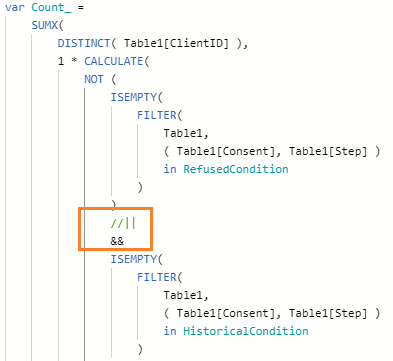Super User

@JustinDoh1 , Create a new column like

New column =
var _1 = countx(filter(Table, [Consent] = "Refused" && [Step]=1 && [client_id] = earlier([Clinet_id])),[Clinet_id])+0
var _2 = countx(filter(Table, [Consent] = "Historical" && [Step]=2 && [client_id] = earlier([Clinet_id])),[Clinet_id])+0
return
if(_1 >0 && _2 >0 , "Yes", "No")
// Or if(_1 >0 && _2 >0 && [Consent] = "Historical" , "Yes", "No")

The one in comment at last in case you need yes on a particular stepContinued Contributor

@amitchandak Thank you so much for your help.

I am getting minor syntax/error message.And how do we interpret the second option ([Consent] = "Historical")?

if(_1 >0 && _2 >0 && [Consent] = "Historical" , "Yes", "No")

Thank you.Announcements#### Exclusive opportunity for Women!

Join us for a free, hands-on Microsoft workshop led by women trainers for women where you will learn how to build a Dashboard in a Day!#### Power Platform Conference-Power BI and Fabric Sessions

Join us Oct 1 - 6 in Las Vegas for the Microsoft Power Platform Conference.Top Solution Authors
Top Kudoed Authors
Users online (1,839)